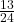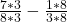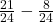How would I find the difference of 7/8 – 1/3 ?

Question

How would I find the difference of 7/8 – 1/3 ?

in progress 0
6 months 2021-08-04T11:46:31+00:00 2 Answers 0 views 0Step-by-step explanation:

We have two fractions and are being asked to subtract them.

7/8 and 1/3 don’t have the same denominator, so to do as so, we need to find the LCM of both denominators, multiply them to that, and do the same for the numerators.

So what is the LCM (Least Common Multiple) of 8 and 3?

24.

Therefore :13/24

Step-by-step explanation:

1. Common denominator:

common denominator = 24

multiply bottom and top so from 8 to 24 is 8×3, so multiply by three and what you do to the bottom you do to the top so,

7/8: 8 x 3 = 24 and 7 x 3 = 21 so the new fraction is 21/24.

Do the same to the other fraction: 1/3 multiply top and bottom by 8 because 3 x 8 = 24.

1/3 = 8/24

Now just subtract: